﻿ Sedimentation Analysis of Galena Concentrate and Predictability of Effective Particle Depth Based on Settling Time and Particle Diameter

### Sedimentation Analysis of Galena Concentrate and Predictability of Effective Particle Depth Based on...

C. I. Nwoye, S. O. Nwakpa, I. D. Adiele, M. A. Allen, S. E. Ede, N. E. IdenyiOPEN ACCESSPEER-REVIEWED

## Sedimentation Analysis of Galena Concentrate and Predictability of Effective Particle Depth Based on Settling Time and Particle Diameter

C. I. Nwoye1,, S. O. Nwakpa1, I. D. Adiele2, M. A. Allen3, S. E. Ede4, N. E. Idenyi5

1Department of Metallurgical and Materials Engineering, NnamdiAzikiwe University, Awka, Nigeria

2Project Development Institute Enugu, Nigeria

3Department of Mechanical Engineering, MichealOkpara University, Umuahia, Nigeria

4Department of Metallurgical and Materials Engineering, Enugu State University of Science & Technology, Enugu Nigeria

5Department of Industrial Physics, Ebonyi State, Abakiliki, Nigeria

### Abstract

Galena particles (concentrate) were analyzed by sedimentation method using conventional technique, appropriate apparatus and reagents. A critical evaluation of the relationship between effective particle depth and a consortium of factorials; settling time and particle diameter was carried out using a derived model which is two-factorial-quadratic in nature. Results of the sedimentation analysis show increased effective depth reached by the particles as a result of decrease in the descending particle diameter, and increased settling time. The validity of derived model; β = 2 x 10-5 γ2 - 5 x 10-4 γ – 1.5 x 10-3 α + 0.2176 is rooted on the core expression β - 1.5 x 10-3 = 2 x 10-5 γ2 - 5 x 10-4 γ + 0.2176 where both sides of the expression are correspondingly approximately equal to 0.2. The model validity was verified through comparative evaluation of the settling rates from experimental and model-predicted results. These settling rates are 0.002 and 0.0025 m/mins. respectively which are in proximate agreement. The standard error incurred in predicting the effective particle depth for each value of settling time & particle diameter considered as evaluated from experiment and derived model are 0.0018 and 0.0027 & 0.0005 and 0.0024 respectively. Maximum deviation of model-predicted results from experiment was less than 3%, implying a confidence applicability level of about 97%.

### At a glance: Figures

12345
Prev Next

• Nwoye, C. I., et al. "Sedimentation Analysis of Galena Concentrate and Predictability of Effective Particle Depth Based on Settling Time and Particle Diameter." Materials Science and Metallurgy Engineering 2.2 (2014): 17-25.
• Nwoye, C. I. , Nwakpa, S. O. , Adiele, I. D. , Allen, M. A. , Ede, S. E. , & Idenyi, N. E. (2014). Sedimentation Analysis of Galena Concentrate and Predictability of Effective Particle Depth Based on Settling Time and Particle Diameter. Materials Science and Metallurgy Engineering, 2(2), 17-25.
• Nwoye, C. I., S. O. Nwakpa, I. D. Adiele, M. A. Allen, S. E. Ede, and N. E. Idenyi. "Sedimentation Analysis of Galena Concentrate and Predictability of Effective Particle Depth Based on Settling Time and Particle Diameter." Materials Science and Metallurgy Engineering 2, no. 2 (2014): 17-25.

 Import into BibTeX Import into EndNote Import into RefMan Import into RefWorks

### 1. Introduction

It has been reported  that processing systems with distribution of particle sizes and shape are produced by proper selection and blending of raw materials with different initial characteristics and by subsequent crushing, grinding, dispersion, classification and granulation. Studies  have been carried out on the packing of coarse (0.37mm) monosize spherical particles using axial vibration. During the experiment, packing was observed to be mostly in an orthorhombic arrangement and packing density of 62.5%. The report concluded that packing density of nearly monosized spherical particles of silica and alumina of colloidal size packed by filter pressing deflocculated slurry is about 60-65%.

The accuracy of particle size data has been reported  to depend somewhat on the sample preparation, the particle shape and technique used for the analysis. It has been reported  that techniques in current use are microscopy, sieving, sedimentation, electrical sensing, laser diffraction and light intensity fluctuation. Sedimentation analysis has been reported  to be more precise and sensitive to the size distribution of clay suspension than electrical sensing technique.

Previous research  revealed that in electrical sensing techniques, the resistance of an electrolyte current path through a narrow orifice between two electrode increases when ceramic particle pass through the orifice. The resistance pulse for a stream of dispersed particle passing through the orifice are converted into the voltage pulse, amplified, scaled and counted electronically.

Basically, sedimentation of particles in a fluid has long been used to characterize particle size distribution. In sedimentation analysis using hydrometer method, spherical particles with a particular density and diameter are released into a viscous fluid to ascertain the velocity and time of settling. In gravitational sedimentation, sedimentation analysis relies on the relationship that exits between settling velocity and particle diameter. Settling velocity is related to the diameter of a spherical particle. The force acting downward on each particle due to its weight in water is given by :(1)

where X is the particle diameter, ρs is the particle density, ρ1 is the liquid density, and g is the acceleration due to gravity.(2)

Equation (2) is a form of the relationship first developed by Stokes in 1851 and is now known as Stokes’ Law. The basic assumptions  used in applying Stokes’ Law to sediment particle suspensions are that: (i) Terminal velocity is attained as soon as settling begins.(ii) Resistance to settling is entirely due to viscosity of the fluid. (iii) Particles are smooth and spherical (iv) There is no interaction between individual particles in the solution.

Stokes`s law  has since been used to determine an unknown distribution of spherical particle size by measuring the time required for the particles to settle at a known distance in a fluid of known viscosity and density. Sedimentation can be either gravitational (1g- force), or centrifugal (many g- forces).

Report  has shown that gravitational sedimentation is normally limited to particles of relatively large size, because the rate of sedimentation for small particles is too low to give a practical analysis time, and because Brownian motion of small particles becomes too large to allow effective settling. The research posited that very small particles (< 0.1 microns) never settle by gravity unless they are extremely dense. This implies that very small particles cannot be measured by gravitational sedimentation. Further research indicated that centrifugal sedimentation involves much smaller particles. High g-force makes sedimentation of small particles much faster than Brownian diffusion, even for very small particles. It was ascertained that when a centrifuge is used, Stokes`s law must be modified to account for the variation in g-force with distance from the center rotation.

It has been shown  that all the parameters except time are constant during centrifugal sedimentation analysis where a centrifuge is running at constant speed and temperature.

Research  has classified the methods of sedimentation analysis into two: integral and differential. The differential method was first reported in 1930  as a viable means of measuring particle sizes. It was reported  that sedimentation instability or streaming results when there is bulk settling of particles in differential sedimentation. The researcher concluded that all information about the particle size distribution could be lost if streaming takes place. Studies [11, 12, 13] have revealed several methods developed to eliminate streaming. Each of these methods was found to be effective because a slight density gradient is formed within the fluid column prior to starting analyses.

Past findings  have shown that Stoke`s law does not accurately describe the sedimentation process if the Reynolds number for the system becomes too high. The Reynolds number increases with larger particles, faster sedimentation rate, and lower fluid viscosity. Based on the foregoing, sedimentation analyses are run at low Reynolds number ((< 0.02), where the deviation from Stokes`s law is less than 0.5%.

The present work is to carry out a sedimentation analysis of galena particle (concentrate) and predictability of effective particle depth based on the settling time and particle diameter.

### 2. Materials and Method

2.1. Beneficiation Process

Solid minerals composed of Galena (PbS) and sphalerite (ZnS) were collected in lumps from deposits in South-eastern Nigeria. The crude Pb-Zn sulphide ore was pulverized and beneficiated (at National Metallurgical Centre, Jos) using sodium silicate, sodium xanthate and methl isobutyl ketone (MIBK) as depressant, collector and frother respectively. The pH of the liquid ore was regulated within 8-13. After the beneficiation process, each batch of the lead sulphide concentrate was homogenized prior to chemical analysis.

2.2. Sedimentation Process

Following beneficiation of the ore, the concentrates were pretreated using a dispersing agent (sodium hexametaphosphate) to remove any gangue materials left. Sedimentation analysis was carried out (at a test temperature of 300C) using the conventional procedure (BS1377:1975, Test 6(B)). Hydrometer type used was casagranda 200C. During the experiment, general corrections were done to facilitate accuracy of results. These corrections are meniscus correction cm, temperature correction Mt and dispersing agent correction X, and water density correction cw. The difference between the two scales readings multiplied by 1000 gives the meniscus correction;

### 3. Results and Discussion

3.1. Model Formulation

Experimental data obtained from research work were used for this work. Computational analysis of the experimental data shown in Table 1, gave rise to Table 2 which indicate that;

#### Table 1. Results of Sedimentation Analysis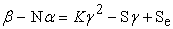(3)

Introducing the values of N, K, S, and Se into equation (3)(4)(5)

Where

(β) = Effective depth of particle (m)

(γ) = Particle diameter (μm)

(α) = Settling time (mins.)

N, K, S and Se are equalizing constants with values 1.5 x 10-3, 2 x 10-5, 5 x 10-4 and 0.2176 respectively (determined using C- NIKBRAN )

### 4. Boundary and Initial Condition

concentrate for the sedimentation analysis was prepared and associated apparatus set according to the conventional procedure (BS 1377:1975, Test 6(B)). Before the start of the leaching process, the hydrometer was assumed to be initially free of attached unwanted bacteria and other micro organism. The effect of oxygen on the process was assumed to be atmospheric since it is an open system process. In all cases, weight of galena used was 50g. The range of the settling time considered: 4-300 seconds. A constant leaching temperature of 25oC and average ore grain size of 150µm were also used. Details of the experimental technique and other process conditions are as presented in the experiment.

A non-zero gradient was assumed for the liquid scalar at the bottom of the particles and for the gas phase at the top of the particles. The sides of the particles were assumed to be symmetries.

4.1. Model validation

The validity of the model is strongly rooted on equation (4) where both sides of the equation are correspondingly approximately equal to 0.2. Table 2 also agrees with equation (4) following the values of β - 1.5 x 10-3 and 2 x 10-5 γ2 - 5 x 10-4 γ + 0.2176 evaluated from the experimental results in Table 1. Furthermore, the derived model was validated by comparing the effective depth of particles predicted by the model and that obtained from the experiment. This was done using various analytical techniques.

4.2. Computational Analysis

Computational analysis of the experimental and model-predicted settling rate was carried out to ascertain the degree of validity of the derived model. This was done by comparing the settling rates obtained by calculations involving experimental results with the model-predicted results.

Settling rate SR (m /mins.) was calculated from the equation;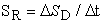(6)

Equation (6) is detailed as(7)

Where

ΔSD = Change in the settling depths SD2, SD1 within a range of settling time: t1 - t2.

Δt = Change in the leaching times t2, t1.

Therefore, a plot of effective depth of particles against settling time as in Figure 1 using experimental results in Table 1, and substitution of points (0.1333, 0.2111) and (5, 0.2234) for (t1, SD1 ) and (t2, SD2) respectively into the mathematical expression in equation (7) gives 0.0025 m/mins as the settling rate of the galena particles during the actual sedimentation process.

Similarly, a plot of effective depth of particles against settling time as in Figure 2 using model-predicted results, and substitution of points (0.1333, 0.2147) and (5, 0.2243) for (t1, SD1 ) and (t2, SD2) respectively into the mathematical expression in equation (7) gives 0.002 m/mins as the model-predicted settling rate of the galena particles. These settling rates evaluated from experiment and derived model indicate proximate agreement and hence validity of the model.

Figure 4. Coefficient of determination between effective depth of particle and settling time as obtained from the experiment
4.3. Statistical Analysis

The standard errors (STEYX) incurred in predicting the effective particle depth for each value of settling time & particle diameter considered as evaluated from experiment and derived model are 0.0018 and 0.0027 & 0.0005 and 0.0024 respectively. The standard errors were evaluated using Microsoft Excel 2003 .

Figure 6. Coefficient of determination between effective depth of particle and particle diameter as obtained from the experiment

The correlations between effective depth of particle and settling time as well as effective depth of particle and particle diameter as obtained from derived model and experimental results were calculated. This was done by considering the coefficients of determination R2 from Figure 1-Figure 4, using the equation;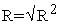(8)

The evaluated correlations are shown in Table 3 and Table 4 for experimental and model-predicted results which are in proximate agreement.

#### Table 4. Comparison of the correlations between extraction rate and final pH of leaching solution as evaluated from experimental and derived model

4.4. Graphical Analysis

Comparative graphical analysis of Figure 8 and Figure 9 shows very close alignment of the curves from model-predicted effective particle depth (MoD) and that of the experiment (ExD). The degree of alignment of these curves is indicative of the proximate agreement between both experimental and model-predicted effective particle depth.

Figure 8. Comparison of effective depths of particles (relative to settling time) as obtained from experiment and derived model
Figure 9. Comparison of effective depths of particles (relative to particle diameter) as obtained from experiment and derived model

Graphical analysis of Figure 8-Figure 10 show very close alignment of the curves from derived model (D-MoD) and experimental (ExD) results of effective particle depth. It is strongly believed that the degree of alignment of these curves is indicative of the proximate agreement between ExD and MoD predicted results. Figure 10 shows a two-factorial dependency of effective particle depth; on settling time and particle diameter. This indicates that for any pre-determined values of settling time and particle diameter within the boundary conditions, the effective depth reached by the particles during descend could be predicted.

Figure 10. Comparison of effective depths of particles (jointly relative to settling time and particle diameter) as obtained from experiment and derived mode
4.5. Deviational Analysis

Comparative analysis of effective particle depth from experiment and derived model revealed deviations on the part of the model-predicted values relative to values obtained from the experiment. This is attributed to the fact that the surface properties of the galena particles and the physiochemical interactions between the particles and the dispersing agent (sodium hexametaphosphate) which played vital roles during the sedimentation process were not considered during the model formulation. This necessitated the introduction of correction factor, to bring the model-predicted effective particle depth to those of the corresponding experimental values.

Deviation (Dn) of model-predicted effective particle depth from that of the experiment is given by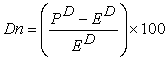(9)

Correction factor (Cr ) is the negative of the deviation i.e(10)

Therefore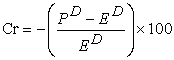(11)

Where

PD = Model-predicted effective particle depth (m)

ED = effective particle depth from experiment (m)

Cr = Correction factor (%)

Dn = Deviation (%).

Figure 11. Comparison of effective depths of particles (relative to deviation of model-predicted results from experimental values)

Introduction of the corresponding values of Cr from equation (11) into the model gives exactly the corresponding experimental effective particle depth: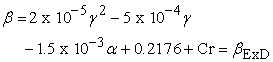(12)

Figure 11 shows that the maximum deviation of the model-predicted effective particle depth from the corresponding experimental values is less than 3% and quite within the acceptable deviation limit of experimental results.

These figures show that the least and highest magnitudes of deviation of the model-predicted effective particle depth (from the corresponding experimental values) are +0.22 and + 2.29% which corresponds to effective particle depths: 0.2227 and 0.2147 m and settling times: 4 and 0.0667 mins. and particle diameters: 1.97 and 14.83 μm respectively.

Figure 13. Variation of model-predicted effective particle depth with associated deviation and correction factor

Comparative analysis of Figure 11-Figure 13 indicates that the orientation of the curve in Figs. 12 and 13 is opposite that of the deviation of model-predicted effective particle depth (Figure 11). This is because correction factor is the negative of the deviation as shown in equations (10) and (11). It is believed that the correction factor takes care of the effects of the surface properties of the galena particles and the physiochemical interactions between the particles and the dispersing agent which played vital roles during the sedimentation process, but were not considered during the model formulation. Figure 9 and Figure 10 indicate that the least and highest magnitudes of correction factor to the model-predicted effective particle depth are - 0.22 and – 2.29%, which corresponds to effective particle depths: 0.2227 and 0.2147 m and settling times: 4 and 0.0667 mins. and particle diameters: 1.97 and 14.83 μm respectively.

It is important to state that the deviation of model predicted results from that of the experiment is just the magnitude of the value. The associated sign preceding the value signifies if the deviation is deficit (negative sign) or surplus (positive sign).

### 5. Conclusion

Results from the sedimentation analysis of galena particles (concentrate) carried out using conventional techniques, appropriate apparatus and reagents indicate that while the settling time increases, the effective depth reached by the particles increases with decrease in the diameter of particles descending. A critical evaluation of the relationship between effective particle depth and a consortium of factorials; settling time and particle diameter resulted in derivation of a model which is two-factorial-quadratic in nature. The validity of derived model; β = 2 x 10-5 γ2 - 5 x10-4 γ – 1.5 x 10-3 α + 0.2176 is rooted on the expression β - 1.5 x 10-3 = 2 x 10-5 γ2 - 5 x 10-4 γ + 0.2176 where both sides of the expression are correspondingly approximately equal to 0.2. Verification of the model validity through evaluation of settling rate gave 0.002 and 0.0025 m/mins. for derived model and experiment respectively. The standard errors (STEYX) incurred in predicting the effective particle depth for each value of settling time & particle diameter considered as evaluated from experiment and derived model are 0.0018 and 0.0027 & 0.0005 and 0.0024 respectively. Maximum deviation of model-predicted results from experiment was less than 3%, implying a confidence applicability level of about 97%.

### References

  Reed, J. (1988).Principles of Ceramic Processing, Wiley Interscience Publication Canada, pp. 460-476.In article  McGeary, R. K. (1961).Mechanical Packing Spherical Particles. American Ceramic Society, pp. 44 10:513-520.In article  David, R.W. (1979). Mechanical Behaviour of Ceramics 1st Edition, Cambridge University Press, pp. 67-78.In article  Barsoum, M. (1997). Fundamentals of Ceramics. McGraw Hill Incorporated, Singapore, pp. 400-410.In article  Singer, F and Singer, S. S. (1963).Industrial Ceramics, University Press Cambridge, pp. 34- 44.In article CrossRef  Glendon, W. G, Dani O. R. Utah State University, Logan, Utah.In article  Stoke, G. G. (1880). Mathematical and Physical Papers. Cambridge University Press, London, pp. 11.In article  CPS Equipment EuropeIn article  Marshall, C. E. (1930). A New Method of Determining the Distribution Curve of Polydisperse Colloidal Systems. Proc., Roy., A 126 pp. 427.In article  Allen, T. (1968). Particle Size Measurement, Chapman and Hall, London, pp 120.In article  Brakke, M. K. (1953). Basic Theory of Particles Size Analysis by Sedimentation. Arch., Biochem., Biophysics., 45:275-290.In article CrossRef  Jones, M. H.(1969). Method and Apparatus for Improved Centrifugal Analysis. U. S. Patent 3, 475,968, November 4.In article  Puhk, H. (1987). Method for Determining Particle Size and/or Distribution. U. S. Patent 4, 699,015, October 13.In article  Nwoye, C. I. (2008). Data Analytical Memory; C-NIKBRAN.In article